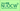# Linear Algebra ILecturer Tetsuya SHIROMIZU, Professor Institute of Liberal Arts & Sciences, 2016 Spring School of Science 2nd year students (2・週１回全１５回)

### Course Overview

"Linearity" is the most basic concept used for numerical methods in many fields of modern science, and linear algebra is the mathematical method that addresses linearity. In the first half of this one-year course, this class aims at mastering the mathematical treatment of matrices, and understanding their concepts. Particularly, this course will focus on understanding a geometrical understanding of matrices through coordinate geometry (planar, spatial), mastering solving systems of linear equations, and understanding the concepts of determinants.

### Course Features

Linear algebra is one of the basic languages used widely in all natural sciences. First, students will learn how matrices and determinants are introduced from familiar planar and spatial vectors. Then, students will gradually get used to matrices and determinants through calculations. Moreover, students will experience how useful they are for solving systems of linear equations.

### Course Schedule

``````1.	Planar and spatial vectors
``````
• By learning the equations, directional vectors, and normal vectors of straight lines and planes, students will develop a geometrical understanding of equations. Also, students will understand the relation between rotations inside a plane and matrices, and the geometrical meaning of determinants.
1. Matrices- Students shall understand the basic concept of matrices and master the rules of operation.
1. Determinants- Students will master basic properties and calculations of determinants. Moreover, students will learn the relationship between nonsingularity and determinants of matrices

2. Elementary operations on matrices and systems of linear equations.- Through elementary operations on matrices, students will understand the concept of rank and their relationship with systems of linear equations. In addition, students will master how to distinguish regular (nonsingular) matrices and how to calculate inverse matrices.

#### Last updated

May 10, 2020

OCW website, and all course materials made public on this site,
may be used under the terms and conditions posted under "About OCW"
© 2020 Nagoya University Open Course Ware